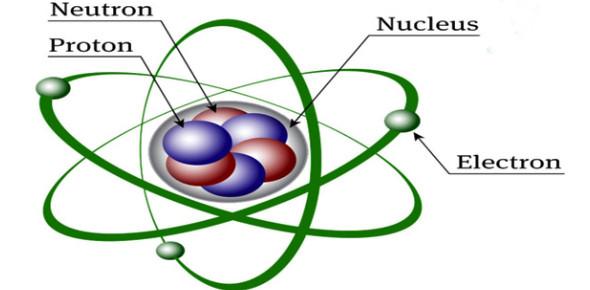Atomic Structure Quiz: Trivia Test!

18 Questions | Total Attempts: 10746SettingsWould you be able to explain atomic structure? It is an atom structure, which consists of a positively charged nucleus surrounded and neutralized by negatively charged electrons circling in orbits at varying distances from the nucleus. The arrangement of electrons contrasts with various chemical elements. The number of electrons is equivalent to the number of protons. You may need to know this information for an exam, and this quiz can come to your rescue.

• 1.
A neutron carries a positive charge.
• A.

True

• B.

False

• 2.
How many atoms of nitrogen are in the formula NH4OH?
• 3.
How many atoms of oxygen are in Sn(SO4)2?
• 4.
Identify the atomic number of the element of Xenon.
• 5.
The atomic number of an atom is determined by the number of
• 6.
A proton has a charge that is equal in force but opposite in charge of each electron.
• A.

True

• B.

False

• 7.
Protons and electrons are about equal in mass.
• A.

True

• B.

False

• 8.
The mass of an atom depends on the number of protons and neutrons in its nucleus.
• A.

True

• B.

False

• 9.
How do you determine the mass number of an atom?
• A.

It is equal to the number of protons

• B.

It is equal to the number of protons plus the number of electrons

• C.

It is equal to the number of protons plus the number of neutrons

• D.

It is equal to the number of electrons plus the number of neutrons

• E.

It is equal to the number of protons minus the number of neutrons

• 10.
Atoms of the same element with different numbers of neutrons are called__________.
• A.

Isotopes

• B.

Ions

• C.

Atoms

• D.

Either isotopes or ions

• E.

None of these are correct

• 11.
In the name carbon-14, the 14 is the _________________.
• A.

Mass number

• B.

Atomic number

• C.

Oxidation number

• D.

Number of electrons

• E.

Number of neutrons

• 12.
An atom that has a different number of electrons and protons is called a(n) _________________.
• 13.
Electrons have a ____________ charge.
• 14.
Neutrons have a ____________ charge.
• 15.
How many protons are in tin-100?
• 16.
How many neutron are in tin-100?
• 17.
Is tin-100 an isotope?
• 18.
What is the charge on an atom with 10 protons and 8 electrons?
Related TopicsBack to top